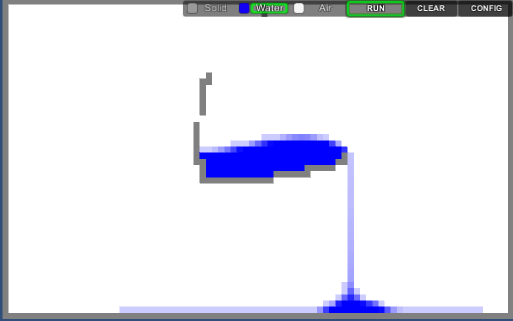Using cellular automata I implemented a simple fluid simulation into the Unity Engine. Each cell in this implementation represents a unit in space containing either fluid, air, or solid mass. For each frame of the simulation a calculation is made attempting to move all the fluid into a state of equilibrium, where the fluid in the surrounding cells roughly equals the fluid of the current cell.

The physics of the simulation are modeled on the basics of fluid motion in a 2D environment, where fluid is first moved down then to the sides and finally up. The core implementation of the simulation is as follows

```            for (int y = 1; y &amp;lt; Row - 1f; ++y)
{
for (int x = 1; x &amp;lt; Column -1f; ++x)
{
curr = caFront[x, y];
flow = 0f;

if (curr.cType != CellType.Solid &amp;amp;&amp;amp; curr.cellMass &amp;gt; 0)
{

rMass = curr.cellMass;

//Below
if (rMass &amp;gt; 0 &amp;amp;&amp;amp; caFront[x, y - 1].cType != CellType.Solid)
{
flow = stableMass(rMass + caFront[x, y - 1].cellMass) - caFront[x, y - 1].cellMass;
if (flow &amp;gt; MinMass) flow *= 0.5f;

flow = Mathf.Clamp(flow, 0f, Mathf.Min(spd, rMass));

caBack[x, y].cellMass -= flow;
caBack[x, y - 1].cellMass += flow;
flowScore += flow;
rMass -= flow;
}

//Left
if (rMass &amp;gt; 0 &amp;amp;&amp;amp; caFront[x + 1, y].cType != CellType.Solid)
{
flow = (curr.cellMass - caFront[x + 1, y].cellMass) * 0.5f;
if (flow &amp;gt; MinMass) flow *= 0.5f;

flow = Mathf.Clamp(flow, 0f, rMass);

caBack[x, y].cellMass -= flow;
caBack[x + 1, y].cellMass += flow;
flowScore += flow;
rMass -= flow;
}

//Right
if (rMass &amp;gt; 0 &amp;amp;&amp;amp; caFront[x - 1, y].cType != CellType.Solid)
{
flow = (curr.cellMass - caFront[x - 1, y].cellMass) * 0.5f;
if (flow &amp;gt; MinMass) flow *= 0.5f;

flow = Mathf.Clamp(flow, 0f, rMass);

caBack[x, y].cellMass -= flow;
caBack[x - 1, y].cellMass += flow;
flowScore += flow;
rMass -= flow;
}

//Above
if (rMass &amp;gt; 0 &amp;amp;&amp;amp; caFront[x, y + 1].cType != CellType.Solid)
{
flow = rMass - stableMass(rMass + caFront[x, y + 1].cellMass);
if (flow &amp;gt; MinMass) flow *= 0.5f;

flow = Mathf.Clamp(flow, 0f, Mathf.Min(spd, rMass));

caBack[x, y].cellMass -= flow;
caBack[x, y + 1].cellMass += flow;
flowScore += flow;
rMass -= flow;
}

}
}
}

The determination of whether to move fluid happens using a calculation averaging the fluid mass of the currently considered neighbor cell and the current cell being processed like so
private float stableMass(float mass)
{
if (mass &amp;lt;= 1f)
return 1f;
else if (mass &amp;lt; 2f * MaxMass + MaxCompress)
return (MaxMass * MaxMass + mass * MaxCompress) / (MaxMass + MaxCompress);
else
return (mass + MaxCompress) * 0.5f;
}

After processing a cell the resulting data is updated to the data structure for the next frame to be drawn. Once all the cells have been updated the data structures for the current and next frame are swapped and the cells are redrawn from new current frame.Tweet

```